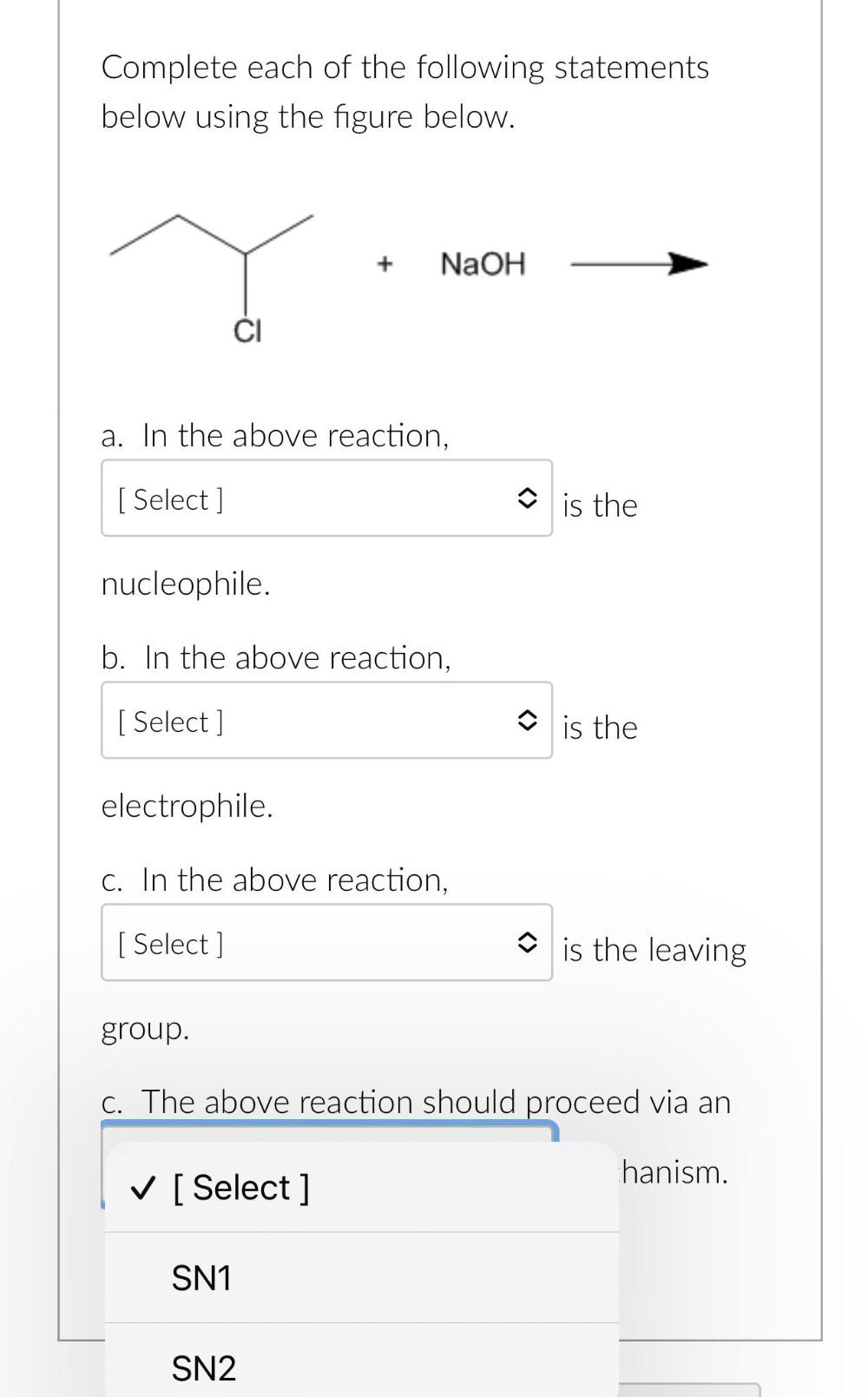Question:

# Complete each of the following statements below using the figure below. + NaOH a. In the above reaction, [ Select ] Ô is the nucComplete each of the following statements below using the figure below. + NaOH a. In the above reaction, [ Select ] Ô is the nucleophile. b. In the above reaction, [ Select ] Ô is the electrophile. C. In the above reaction, [ Select] is the leaving group. C. The above reaction should proceed via an hanism. ✓ [ Select ] SN1 SN2